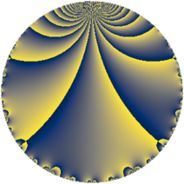Properties

 Label 32.3.dLevel $32$ Weight $3$ Character orbit 32.d Rep. character $\chi_{32}(15,\cdot)$ Character field $\Q$ Dimension $1$ Newform subspaces $1$ Sturm bound $12$ Trace bound $0$

Related objects

Defining parameters

 Level: $$N$$ $$=$$ $$32 = 2^{5}$$ Weight: $$k$$ $$=$$ $$3$$ Character orbit: $$[\chi]$$ $$=$$ 32.d (of order $$2$$ and degree $$1$$) Character conductor: $$\operatorname{cond}(\chi)$$ $$=$$ $$8$$ Character field: $$\Q$$ Newform subspaces: $$1$$ Sturm bound: $$12$$ Trace bound: $$0$$

Dimensions

The following table gives the dimensions of various subspaces of $$M_{3}(32, [\chi])$$.

Total New Old
Modular forms 12 3 9
Cusp forms 4 1 3
Eisenstein series 8 2 6

Trace form

 $$q + 2 q^{3} - 5 q^{9} + O(q^{10})$$ $$q + 2 q^{3} - 5 q^{9} - 14 q^{11} + 2 q^{17} + 34 q^{19} + 25 q^{25} - 28 q^{27} - 28 q^{33} - 46 q^{41} - 14 q^{43} + 49 q^{49} + 4 q^{51} + 68 q^{57} + 82 q^{59} - 62 q^{67} - 142 q^{73} + 50 q^{75} - 11 q^{81} - 158 q^{83} + 146 q^{89} - 94 q^{97} + 70 q^{99} + O(q^{100})$$

Decomposition of $$S_{3}^{\mathrm{new}}(32, [\chi])$$ into newform subspaces

Label Dim. $$A$$ Field CM Traces $q$-expansion
$a_{2}$ $a_{3}$ $a_{5}$ $a_{7}$
32.3.d.a $1$ $0.872$ $$\Q$$ $$\Q(\sqrt{-2})$$ $$0$$ $$2$$ $$0$$ $$0$$ $$q+2q^{3}-5q^{9}-14q^{11}+2q^{17}+34q^{19}+\cdots$$

Decomposition of $$S_{3}^{\mathrm{old}}(32, [\chi])$$ into lower level spaces

$$S_{3}^{\mathrm{old}}(32, [\chi]) \cong$$ $$S_{3}^{\mathrm{new}}(8, [\chi])$$$$^{\oplus 3}$$